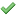MTHS110 Quantitative Skills with Applications

Updated: 08 July 2019
Credit Points 6
Offering
Location Teaching Period Mode of Study
Armidale OUA Trimester 2 Online
Armidale Trimester 1 Online
Armidale Trimester 1 On Campus
Armidale Trimester 2 Online
Armidale Trimester 2 On Campus
Intensive School(s)

Intensive schools are for students enrolled in Online Mode only, unless specified in the notes.

Start Finish Attendance Notes
01 May 2019 03 May 2019 Non-Mandatory Trimester 1 Intensive School
02 September 2019 04 September 2019 Non-Mandatory Trimester 2 Intensive School
Supervised Exam There is a supervised exam at the end of the teaching period in which you are enrolled. The paper-based exam will be held at an established exam venue, and coordinated by UNE Exams Unit.
Pre-requisites None
Co-requisites None
Restrictions MATH120
Notes

Students contemplating enrolment in MTHS110 who are not familiar with the content of General Mathematics in NSW or its equivalent should contact staff in the School of Science and Technology at mathsenquiries@une.edu.au before enrolling. In your e-mail please state your course of study and your mathematics background.

Combined Units None
Coordinator(s) Timothy Schaerf (timothy.schaerf@une.edu.au)
Unit Description

Mathematical models appear throughout the natural and social sciences. For example, growth of both cell populations and money can be modelled using exponential functions. In this unit, students will learn how to develop, understand and apply mathematical models, and see how the universality of mathematics connects seemingly disparate fields. Emphasis is placed on developing good mathematical intuition as well as technical problem-solving, allowing students to immediately apply the knowledge learned in this unit or continue on to the calculus-based mathematics units. This unit follows the Introduction to Quantitative Skills unit or NSW HSC Mathematics or equivalent.

Topics covered include functions and graphing review; exponential, logarithmic, and inverse functions and equations; trigonometric functions and equations (a unit circle approach); solving systems of linear equations using matrices; and sequences and series: solving first-order recurrence relations, arithmetic progressions, geometric progressions.

Prescribed Material
Mandatory

Text(s):

Note: Students are expected to purchase prescribed material. Please note that textbook requirements may vary from one teaching period to the next.

Precalculus: Mathematics for Calculus, International Metric Edition

ISBN: 9781305999985
Stewart, J., Redlin, L. and Watson, S., Cengage 7th ed. 2016

Note: The 6th ed. (ISBN: 9781305071759) is also acceptable.

Text refers to: Trimester 1 and 2, On Campus and Online

Disclaimer Unit information may be subject to change prior to commencement of the teaching period.
Assessment
Must
Complete
Title Exam Length Weight Mode No. WordsAssessment 1 4%
Assessment Notes

Mathematical calculations and problem solving.

Relates to Learning Outcomes (LO)

LO: 1, 2, 3, 4, 5, 6Assessment 2 5%
Assessment Notes

Mathematical calculations and problem solving.

Relates to Learning Outcomes (LO)

LO: 1, 2, 3, 4, 5, 6Assessment 3 4%
Assessment Notes

Mathematical calculations and problem solving.

Relates to Learning Outcomes (LO)

LO: 1, 2, 3, 4, 5, 6Assessment 4 5%
Assessment Notes

Mathematical calculations and problem solving.

Relates to Learning Outcomes (LO)

LO: 1, 2, 3, 4, 5, 6Assessment 5 4%
Assessment Notes

Mathematical calculations and problem solving.

Relates to Learning Outcomes (LO)

LO: 1, 2, 3, 4, 5, 6Assessment 6 5%
Assessment Notes

Mathematical calculations and problem solving.

Relates to Learning Outcomes (LO)

LO: 1, 2, 3, 4, 5, 6Assessment 7 4%
Assessment Notes

Mathematical calculations and problem solving.

Relates to Learning Outcomes (LO)

LO: 1, 2, 3, 4, 5, 6Assessment 8 5%
Assessment Notes

Mathematical calculations and problem solving.

Relates to Learning Outcomes (LO)

LO: 1, 2, 3, 4, 5, 6Assessment 9 4%
Assessment Notes

Mathematical calculations and problem solving.

Relates to Learning Outcomes (LO)

LO: 1, 2, 3, 4, 5, 6Final Examination 2 hrs 15 mins 60%
Assessment Notes

It is mandatory to pass this component in order to pass the unit.

Relates to Learning Outcomes (LO)

LO: 1, 2, 3, 4, 5, 6

Learning Outcomes (LO) Upon completion of this unit, students will be able to:
1. graph and apply exponential and logarithmic functions;
2. solve exponential and logarithmic equations;
3. graph and apply trigonometric functions and solve trigonometric equations;
4. solve systems of linear equations using matrices;
5. solve first-order recurrence relations, and
6. apply arithmetic and geometric sequences and series.